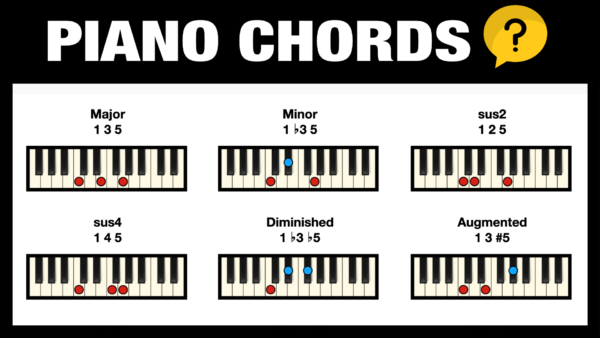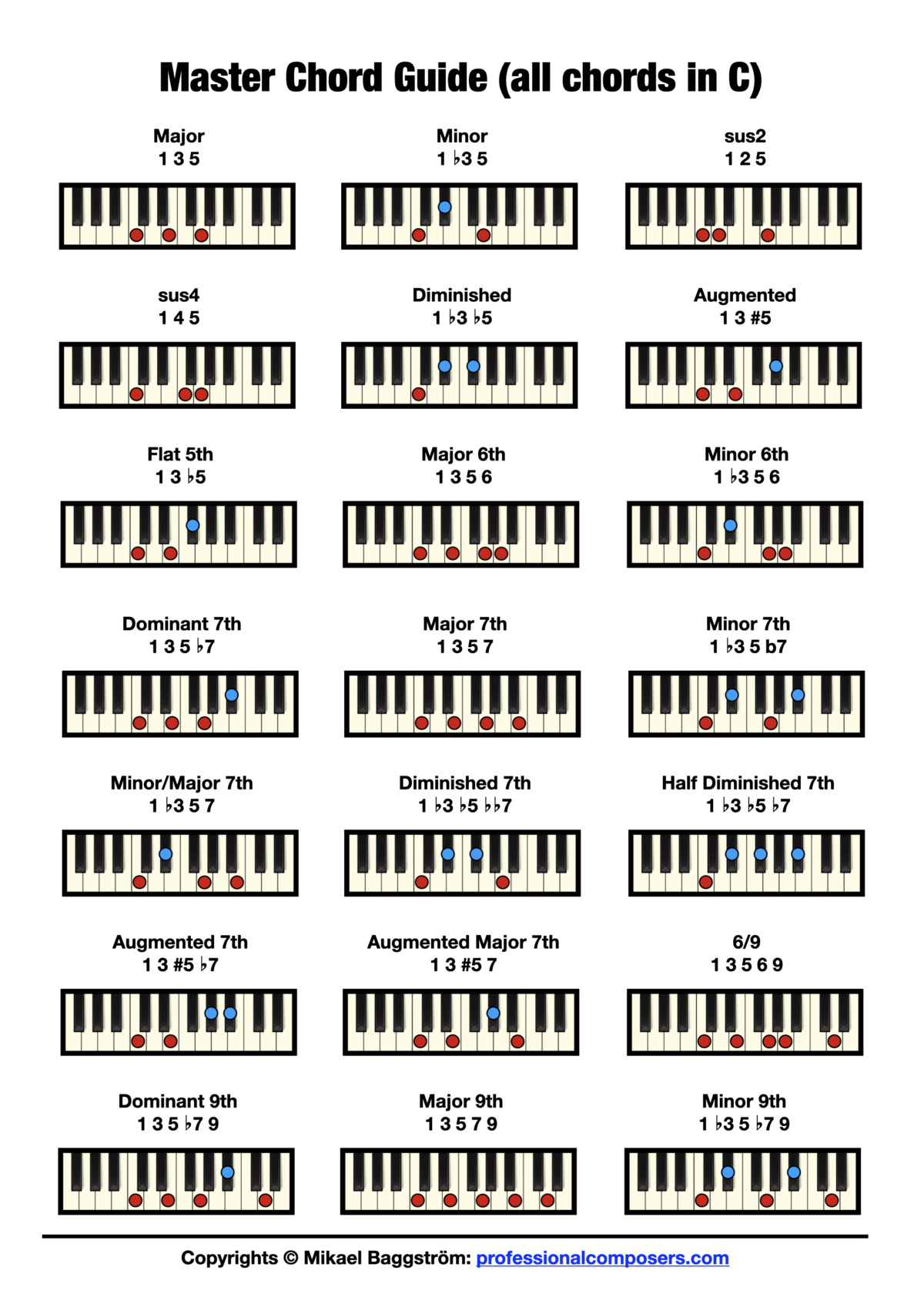Do you want a complete piano chord chart for every single chord you need for music composition?

I have created a complete chart with the 21 most fundamental chords in music, from standard triads up to 9th chord.

You can choose if you want to print it out, or simply bookmark this web page for reference.

# Piano Chord Chart – Quick Guide

First, here’s a list of the chord formula for each chord type.

• major = 1 + 3 + 5
• minor = 1 + b3 + 5
• sus2 = 1 + 2 + 5
• sus4 = 1 + 4 + 5
• diminished = 1 + b3 + b5
• augmented = 1 + 3 + #5
• flat 5th = 1 + 3 + b5
• major 6th = 1 + 3 + 5 + 6
• minor 6th = 1 + b3 + 5 + 6
• dominant 7th = 1 + 3 + 5 + b7
• major 7th = 1 + 3 + 5 + 7
• minor 7th = 1 + b3 + 5 + b7
• minor/major 7th = 1 + b3 + 5 + 7
• diminished 7th = 1 + b3 + b5 + bb7
• half diminished 7th = 1 + b3 + b5 + b7
• augmented 7th = 1 + 3 + #5 + b7
• augmented major 7th = 1 + 3 + #5 + 7
• 6/9 = 1 + 3 + 5 + 6 + 9
• dominant 9th = 1 + 3 + 5 + b7 + 9
• major 9th = 1 + 3 + 5 + 7 + 9
• minor 9th = 1 + b3 + 5 + b7 + 9

# Free Piano Chord Chart

Here’s a complete chord chart for all these 21 fundamental chords on piano (in standard root position) and using “C” as the root note for each chord in the illustrations. Click the image to open in a new tab (for printing out or downloading).